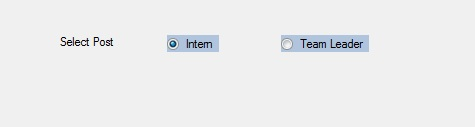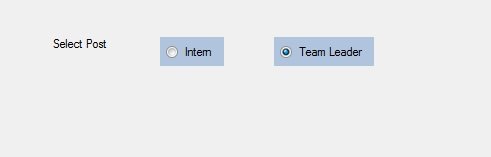Related Articles
• Last Updated : 30 Jun, 2019

In Windows Forms, RadioButton control is used to select a single option among the group of the options. For example, select your gender from the given list, so you will choose only one option among three options like Male or Female or Transgender. In Windows Forms, you are allowed to adjust the padding of the RadioButton using the Padding Property of the RadioButton. Here, padding is the space between the content and the boundaries of the RadioButton. You can set this property in two different ways:

1. Design-Time: It is the easiest way to adjust the padding of the RadioButton as shown in the following steps:

• Step 1: Create a windows form as shown in the below image:
Visual Studio -> File -> New -> Project -> WindowsFormApp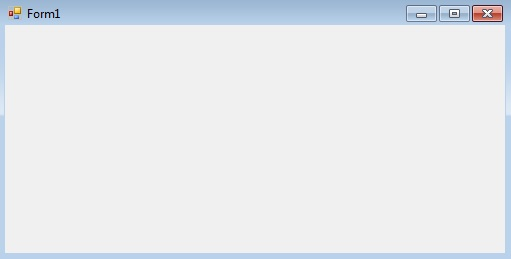• Step 2: Drag the RadioButton control from the ToolBox and drop it on the windows form. You are allowed to place a RadioButton control anywhere on the windows form according to your need.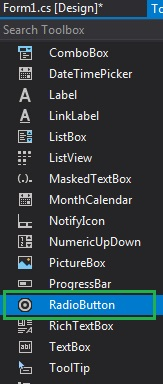• Step 3: After drag and drop you will go to the properties of the RadioButton control to adjust the padding of RadioButton.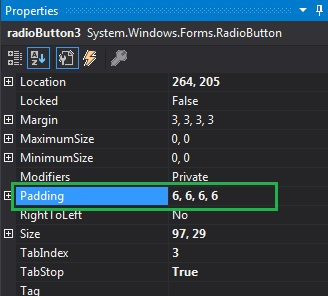Output: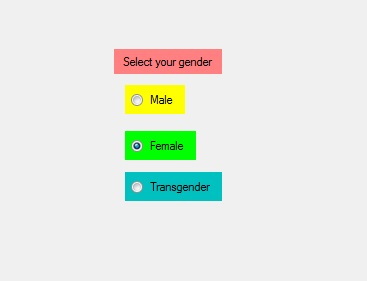2. Run-Time: It is a little bit trickier than the above method. In this method, you can adjust the padding of the RadioButton control programmatically with the help of given syntax:

`public System.Windows.Forms.Padding Padding { get; set; }`

• Step 1: Create a radio button using the RadioButton() constructor is provided by the RadioButton class.
```// Creating radio button
```
```// Setting the padding of the radio button
```
```// Add this radio button to the form
```

Example:

 `using` `System; ` `using` `System.Collections.Generic; ` `using` `System.ComponentModel; ` `using` `System.Data; ` `using` `System.Drawing; ` `using` `System.Linq; ` `using` `System.Text; ` `using` `System.Threading.Tasks; ` `using` `System.Windows.Forms; ` ` `  `namespace` `WindowsFormsApp23 { ` ` `  `public` `partial` `class` `Form1 : Form { ` ` `  `    ``public` `Form1() ` `    ``{ ` `        ``InitializeComponent(); ` `    ``} ` ` `  `    ``private` `void` `Form1_Load(``object` `sender, EventArgs e) ` `    ``{ ` `        ``// Creating and setting label ` `        ``Label l = ``new` `Label(); ` `        ``l.AutoSize = ``true``; ` `        ``l.Location = ``new` `Point(176, 40); ` `        ``l.Text = ``"Select Post"``; ` ` `  `        ``// Adding this label to the form ` `        ``this``.Controls.Add(l); ` ` `  `        ``// Creating and setting the ` `        ``// properties of the RadioButton ` `        ``RadioButton r1 = ``new` `RadioButton(); ` `        ``r1.AutoSize = ``true``; ` `        ``r1.Text = ``"Intern"``; ` `        ``r1.Location = ``new` `Point(286, 40); ` `        ``r1.BackColor = Color.LightSteelBlue; ` `        ``r1.Padding = ``new` `Padding(6, 6, 6, 6); ` ` `  `        ``// Adding this label to the form ` `        ``this``.Controls.Add(r1); ` ` `  `        ``// Creating and setting the  ` `        ``// properties of the RadioButton ` `        ``RadioButton r2 = ``new` `RadioButton(); ` `        ``r2.Text = ``"Team Leader"``; ` `        ``r2.Location = ``new` `Point(400, 40); ` `        ``r2.AutoSize = ``true``; ` `        ``r2.BackColor = Color.LightSteelBlue; ` `        ``r2.Padding = ``new` `Padding(6, 6, 6, 6); ` ` `  `        ``// Adding this label to the form ` `        ``this``.Controls.Add(r2); ` `    ``} ` `} ` `} `

Output: